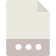Original Post
5th Dan Black Belt
Join Date: Nov 2009
Posts: 4,276
Random useless (or not) mini-scripts thread
For any bits of scripting that don't belong anywhere else. Feel free to share them here.
Newbie scripts are also accepted.

To get things started:

Having been learning how to work with math.cos and math.sin, I have achieved some wonderful results:
lua code:
``````len = 0
ang = 0
w,h = get_window_size()
angtbl = {}

while len <= w do
table.insert(angtbl,{x=(w/2)+(len*math.cos(ang)), y=(h/2)+(len*math.sin(ang))})
len = len + 0.1
ang = ang+0.01
end

set_color(0,0,0,1)circletest.lua (423 Bytes, 83 views)